• 算法--迭代法 万次阅读 多人点赞
2018-10-24 18:52:32

## 迭代法

迭代法（Iteration）是一种不断用变量的旧值递推出新值的解决问题的方法。迭代算法是用计算机解决问题的一种基本方法，一般用于数值计算。累加、累乘都是迭代算法的基础应用。典型案例：牛顿迭代法”。

步骤：

• 确定迭代模型：分析得出前一个（或几个）值与其下一个值的迭代关系数学模型；
• 建立迭代关系式
• 对迭代过程进行控制

经典案例：

示例： 斐波那契数列：1、1、2、3、5、8、13、21、34

function fibonacci(n) {
let a = 1, b = 1, c = 1
for(let i = 2; i <= n; i++) {
c = a + b
a = b
b = c
}
return c
}


对于斐波那契数列，当n趋于无穷时，数列最后的两项的商 (xn-1/xn) 趋于黄金分割数0.618

示例： 最大公约数，采用辗转相除法（欧几里得算法）

定理：两个整数的最大公约数等于其中较小的那个数，和两数相除余数的最大公约数。

gcd(a, b) = gcd(a, a mod b)

function gcd (a, b) {
if (a < b) {
[a, b] = [b, a]
}
let temp
while (b > 0) {
temp = a % b
a = b
b = temp
}
return a
}


示例： 牛顿迭代法

一种在实数域和复数域上近似求解方程的方法，其比一般的迭代法有更高的收敛速度。首先，选择一个接近函数 f(x) 零点的点，如图为
( x n , f ( x n ) ) (x_n, f(x_n))
计算相应的切线斜率
f ′ ( x n ) {f^{'}(x_n)} k = t a n α = y 2 − y 1 x 2 − x 1 k = tan\alpha = \frac{y_2 - y_1}{x_2 - x_1}
得到如下方式
y = f ( x n ) + f ′ ( x n ) ( x n + 1 − x n ) y = f(x_n) + f'(x_n)(x_{n+1} - x_n)
和 x 轴的交点坐标，也就是下面方式的解：
f ( x n ) + f ′ ( x n ) ( x n + 1 − x n ) = 0 f(x_n) + f'(x_n)(x_{n+1} - x_n) = 0
通常 x n + 1 x_{n+1} 会比 x n x_n 更接近方程的解，接下来继续迭代，直到达到要求的精度即可。

x n + 1 = x n − f ( x n ) f ′ ( x n ) \mathbb{x_{n+1} = x_n - \frac{f(x_n)}{f^{'}(x_n)}}
例： a x 3 + b x 2 + c x + d = 0 ax^3 + bx^2 + cx + d = 0 方程的根，系数分别是1，2，3，4。求x在1附近的一个实根。

function f(a, b, c, d) {
let x0, x1 = 1, f0, f1
do {
x0 = x1
f0 = a * Math.pow(x0, 3) + b * Math.pow(x0, 2) + c * x0 + d
// 求导后函数
f1 = 3 * a * Math.pow(x0, 2) + 2 * b * x0 + c
x1 = x0 - f0/f1
} while (Math.abs(x1 - x0) >= Math.pow(Math.E, -4))
return x1
}


例：求根号x的近似值
x 2 = n x 2 − n = 0 f ( x ) = x 2 − n x^2 = n\\ x^2 - n = 0\\ f(x) = x^2 - n
我想求根号2等于多少，我猜测值为4（首先随便猜一个近似值x），根据牛顿迭代定律： x n + 1 = x − x 2 − n 2 x = 1 2 ( x + n x ) x_{n+1} = x - \frac{x^2 - n}{2x} = \frac{1}{2}(x + \frac{n}{x})
1 2 ( 4 + 2 4 ) = 2.25 1 2 ( 2.25 + 2 2.25 ) = 1.56944 1 2 ( 1.56944 + 2 1.56944 ) = 1.42189 1 2 ( 1.42189 + 2 1.42189 ) = 1.41423 \frac{1}{2}(4 + \frac{2}{4}) = 2.25\\ \frac{1}{2}(2.25 + \frac{2}{2.25}) = 1.56944\\ \frac{1}{2}(1.56944 + \frac{2}{1.56944}) = 1.42189\\ \frac{1}{2}(1.42189 + \frac{2}{1.42189}) = 1.41423

function mySqrt (num) {
let x0, x1 = 4, f0, f1
do {
x0 = x1
f0 = Math.pow(x0, 2) - num
// 求导后函数
f1 = 2 * x0
x1 = x0 - f0/f1
} while (Math.abs(x1 - x0) >= Math.pow(Math.E, -4))
return x1
}


引深：

物体直线运动时，路程 s 与时间 t 的函数关系为 s = f ( t ) s = f(t) ，且 f ( t ) f(t) t = t 0 t = t_0 时的导数 f ′ ( t 0 ) f'(t_0) 存在；则在物理上， f ′ ( t 0 ) f'(t_0) 表示物体在时刻 $t_0$ 的瞬时速度 v ( t ) v{(t)} ，而 v ′ ( t ) v'{(t)} 为加速度，即时间 f ’ ′ ( t ) f’'(t) 为加速度！

更多相关内容
• 马尔可夫决策过程的值迭代算法 该存储库的内容作为计算机科学理学硕士课程的学生要求的概率图形模型课程的一项分配项目。 这段代码的版本中提供的所有资源都是从您可以在参考部分找到的类书中获得的。 算法和信息的...
• 随机清洁机器人的基于模型的值迭代算法。 这段代码是值迭代算法的一个非常简单的实现，对于强化学习和动态规划领域的初学者来说，它是一个有用的起点。 随机清洁机器人 MDP：清洁机器人必须收集用过的罐子，还必须为...matlab
• 值迭代（Bellman 1957）：也称为反向归纳，不使用π函数； 而是在需要时在V（s）内计算π（s）的值。 将π（s）的计算代入V（s）的计算可得出组合步骤。 在下面查看有关如何计算效用的示例（有关更多详细信息，请...
• 提出一种基于拓扑序列更新的值迭代算法，利用状态之间的迁移关联信息，将任务模型的有向图分解为一系列规模较小的强连通分量，并依据拓扑序列对强连通分量进行更新。在经典规划问题Mountain Car和迷宫实验中的结果...强化学习 拓扑序列
• 资源名：在matlab平台上，针对多周期报童问题，采用值迭代算法、策略迭代算法和强化学习算法求解MDP模型的实例 资源类型：matlab项目全套源码 源码说明： 全部项目源码都是经过测试校正后百分百成功运行的，如果您...
• 策略迭代算法函数迭代算法 文章目录1. 回顾与引言2. 思路介绍3. 策略评估算法3. 策略优化算法4. 策略迭代算法函数迭代算法5. 代码实现6. 强化学习与最优控制 1. 回顾与引言 上一章中介绍了马尔科夫决策过程...

策略迭代算法和值函数迭代算法

## 1. 回顾与引言

大家如果不了解马尔科夫决策过程可以先阅读这篇文章：https://blog.csdn.net/qq_33302004/article/details/115027798
上一篇文章中介绍了马尔科夫决策过程（MDP），也介绍了状态值函数和行为-状态值函数的计算方法。由此我们已经完成了对强化学习问题的建模过程，我们知道强化学习就是寻找一个最优策略 π \pi ，保证一个已知的MDP ( S , A , P , r , γ ) (S, A, P, r, \gamma) 的累计回报期望最大，也就是：
π = arg max ⁡ π ∫ R ( τ ) p π ( τ ) d τ \pi = \argmax_\pi \int {R(\tau)p_\pi(\tau)} d\tau
我们把已知状态转移概率 P P 的问题有模型问题，把未知 P P 的问题叫做无模型问题，由此最优化MDP的方法可分为基于模型的动态规划方法和基于无模型的强化学习方法，如下图所示：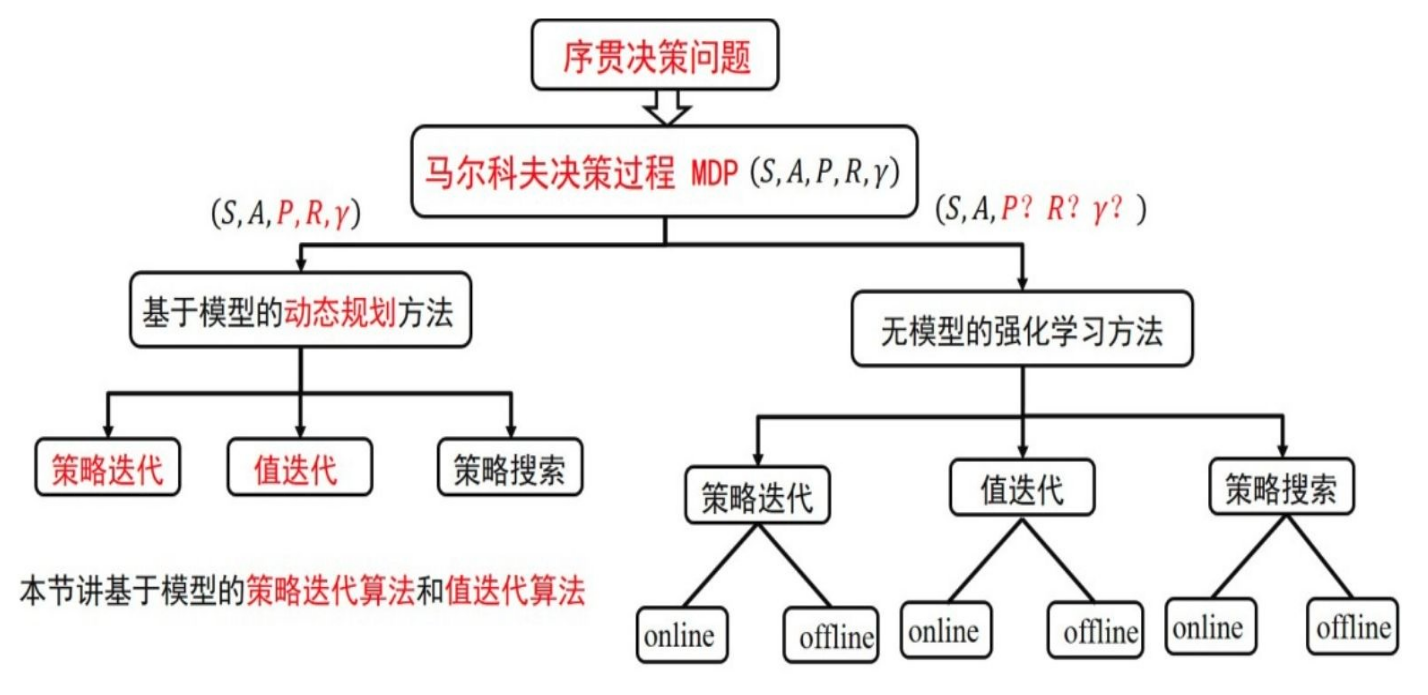由图中可知，这两种方法都包括策略迭代算法、值函数迭代算法、策略搜索算法。本文将介绍基于模型的策略迭代算法值函数迭代算法

## 2. 思路介绍

先不考虑策略迭代或者值函数迭代的概念，来回顾一下我们要解决的问题。在序贯决策问题中，我们知道全部的状态S、可以采用的全部动作A，还知道在状态S下采用动作A会转移到什么状态S‘（P），以及对应的反馈R和损失因子 γ \gamma 。我们现在我们需要考虑两个问题：

1. 如何产生一个策略 π \pi ，也就是： a = π ( s ) a = \pi(s) => 策略优化
2. 如何评价一个策略 π \pi 。 => 策略评估

我们手上有两个武器，状态值函数和行为-状态值函数：
ν π ( s ) = ∑ a ∈ A π ( a ∣ s ) ( R s a + γ ∑ s ′ ∈ S P s s ′ a ν π ( s ′ ) ) \nu_\pi(s) = \sum_{a\in A} \pi(a|s) \left( R_s^a + \gamma\sum_{s' \in S}P_{ss'}^a\nu_\pi(s') \right)
q π ( s , a ) = R s a + γ ∑ s ′ ∈ S P s s ′ a ν π ( s ′ ) q_\pi(s,a) = R_s^a + \gamma\sum_{s' \in S}P_{ss'}^a\nu_\pi(s')
通过状态值函数，我们可以评估策略 π \pi 当前的状态价值分布是如何的，通过行为-状态值函数，我们可以知道在当前状态下采用哪种动作更好，从而优化策略 π \pi

## 3. 策略评估算法

策略评估算法就是在已知一个策略 π \pi 的情况下，如何计算出每个状态的值函数。解决方案是采用“高斯-赛尔德迭代算法”求解状态值函数的迭代公式：
ν π ( s ) = ∑ a ∈ A π ( a ∣ s ) ( R s a + γ ∑ s ′ ∈ S P s s ′ a ν π ( s ′ ) ) \nu_\pi(s) = \sum_{a\in A} \pi(a|s) \left( R_s^a + \gamma\sum_{s' \in S}P_{ss'}^a\nu_\pi(s') \right)
在上面这个式子中出了状态值函数外，所有变量都是已知的，所以本质上就是一个解方程的过程。不理解“高斯-赛尔德迭代算法”的话，可以简单理解为： x = f ( x ′ ) x=f(x') ，本次的 x x 可以用上一次计算的 x ′ x' 求得，并且通过无限迭代最终会收敛，收敛处的 x x 值就是方程组的解。

策略评估算法的伪代码如下：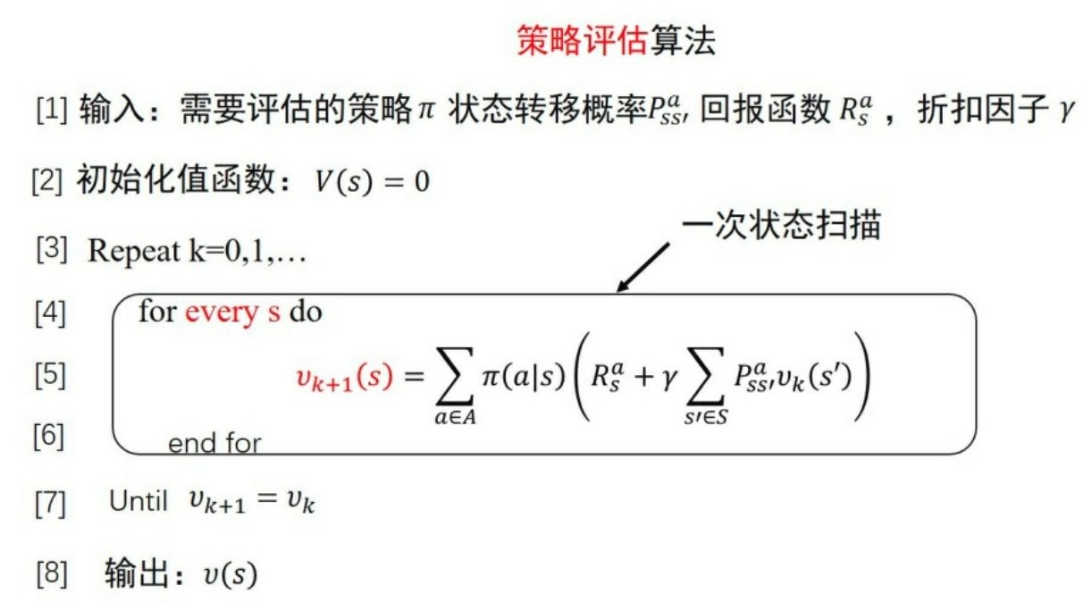我们来举个例子，如下图所示为网格世界，其状态空间为S={1,2,…,14}，动作空间为A={东,南,⻄,北}，回报函数为r≡-1，需要评估的策略为均匀随机策略，也就是东南西北选择的概率都为0.25。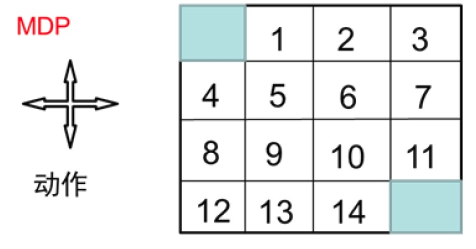迭代0次、1次、2次、3次后每个状态的值函数的值如下图所示：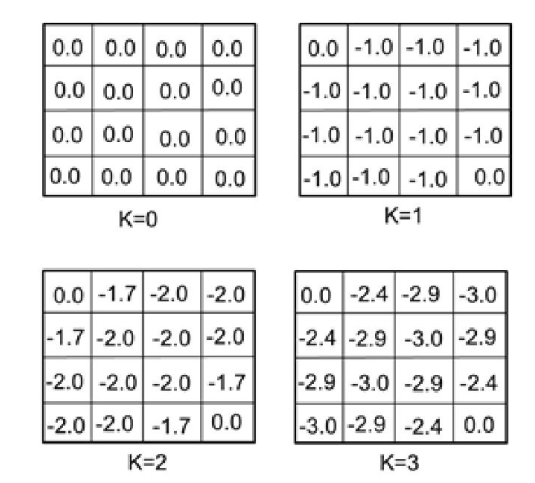状态1，第二次迭代的值函数的值计算过程如下：
v 2 ( 1 ) = 0.25 ( − 1 − 1 ) + 0.25 ( − 1 − 1 ) + 0.25 ( − 1 − 1 ) + 0.25 ( − 1 − 0 ) = − 1.75 ≈ − 1.7 \begin{aligned} v_2(1) &= 0.25(-1-1)+ 0.25(-1-1)+ 0.25(-1-1)+ 0.25(-1-0) \\ &= -1.75\approx-1.7 \end{aligned}
状态1，第三次迭代的值函数的值计算过程如下：
v 3 ( 1 ) = 0.25 ( − 1 − 1.7 ) + 0.25 ( − 1 − 2 ) + 0.25 ( − 1 − 2 ) + 0.25 ( − 1 − 0 ) = − 2.425 ≈ − 2.4 \begin{aligned} v_3(1) &= 0.25(-1-1.7)+ 0.25(-1-2)+ 0.25(-1-2)+ 0.25(-1-0) \\ &= -2.425\approx-2.4 \end{aligned}

## 3. 策略优化算法

现在我们来解决如何改善策略的问题。很自然的解决方案是采用贪心的策略来解决这个问题，也就是在每个状态都采用行为-状态值函数最大的动作，如下图所示：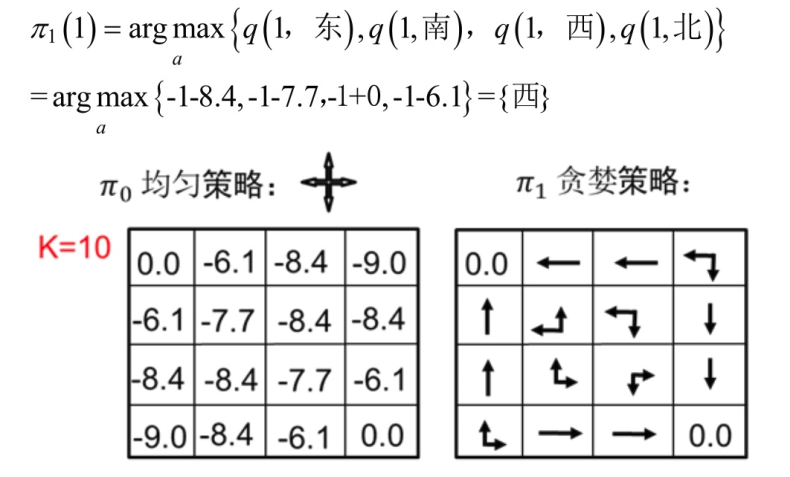## 4. 策略迭代算法和值函数迭代算法

所谓策略迭代是指先初始化策略、然后在每个策略下计算出收敛的状态值（采用策略评估算法），而后根据计算出的值使用策略优化算法修改策略，如此迭代，直到策略收敛，就作为最优策略，伪代码如下：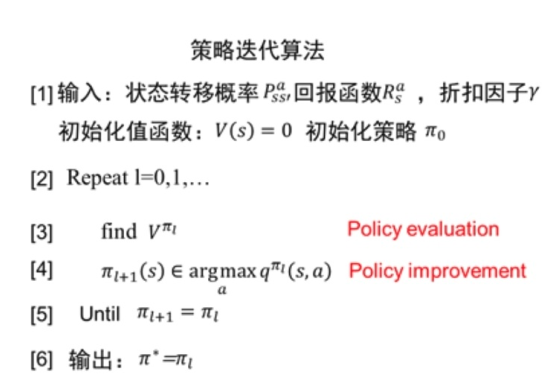值函数迭代算法也是先初始化策略，而后在每次更新状态的值的时候，直接采用当前状态下能够产生的最大值更新值（而不是向策略迭代算法中那样采用均值），直到值收敛后，采用一次策略优化获得最终的策略，伪代码如下：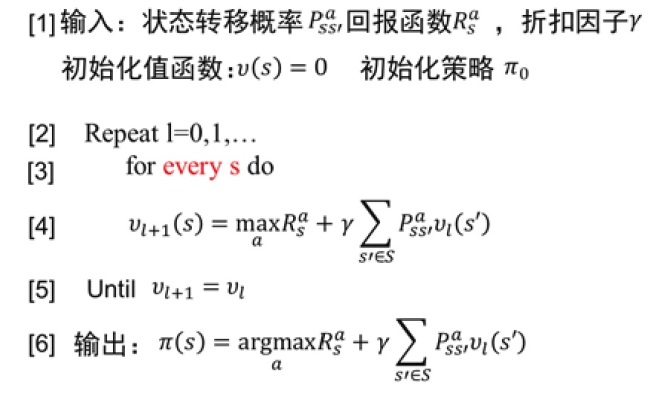## 5. 代码实现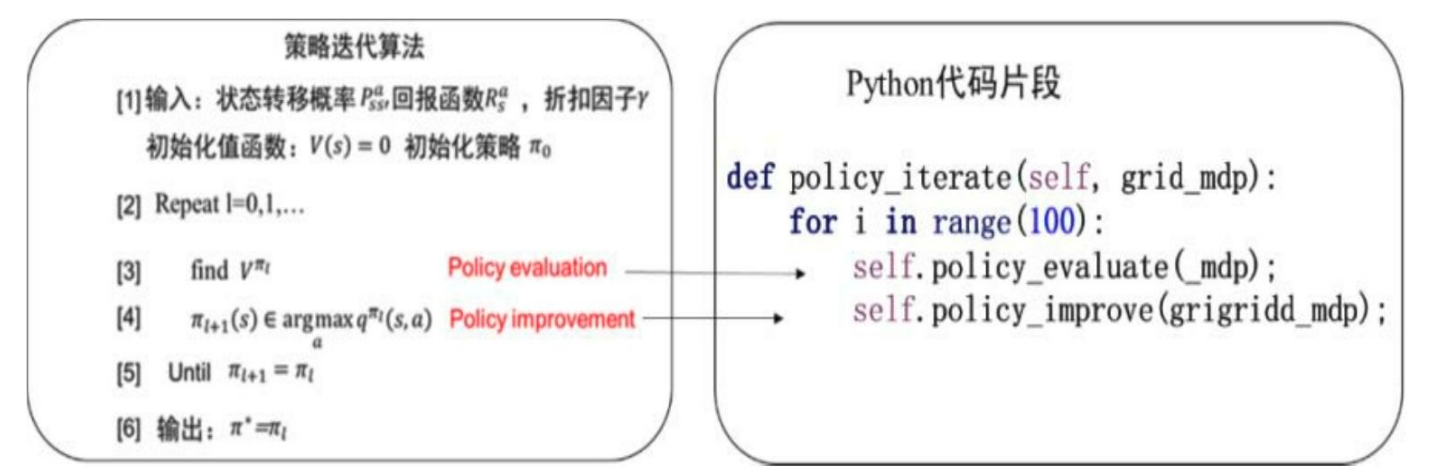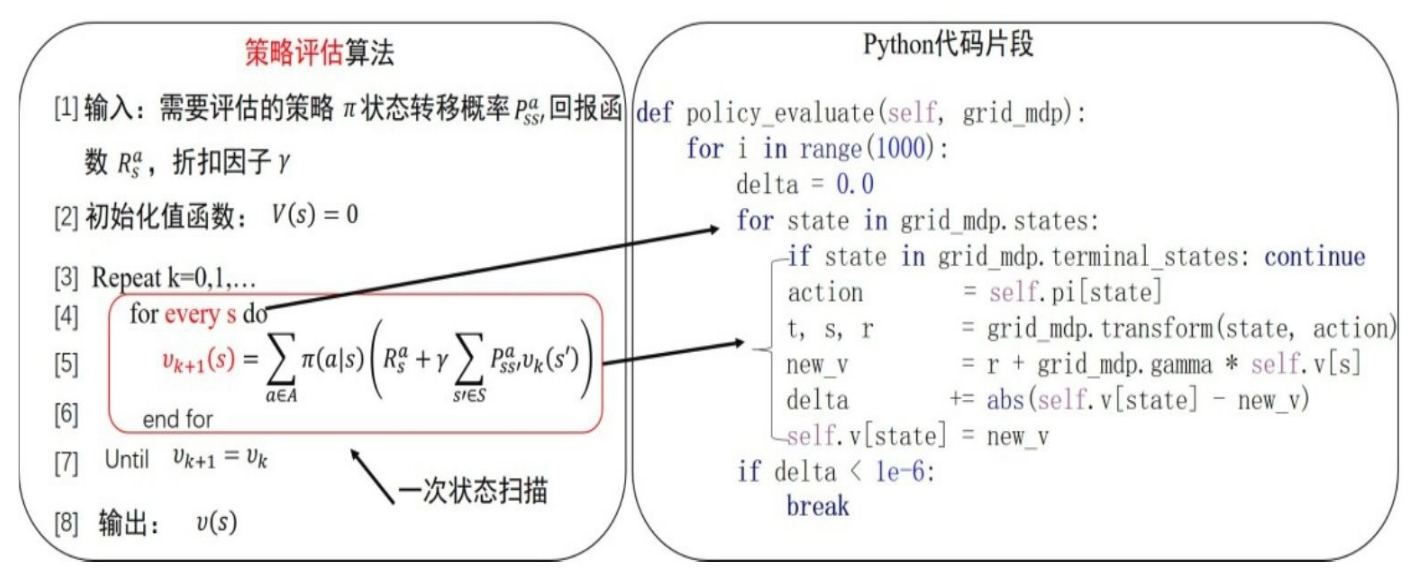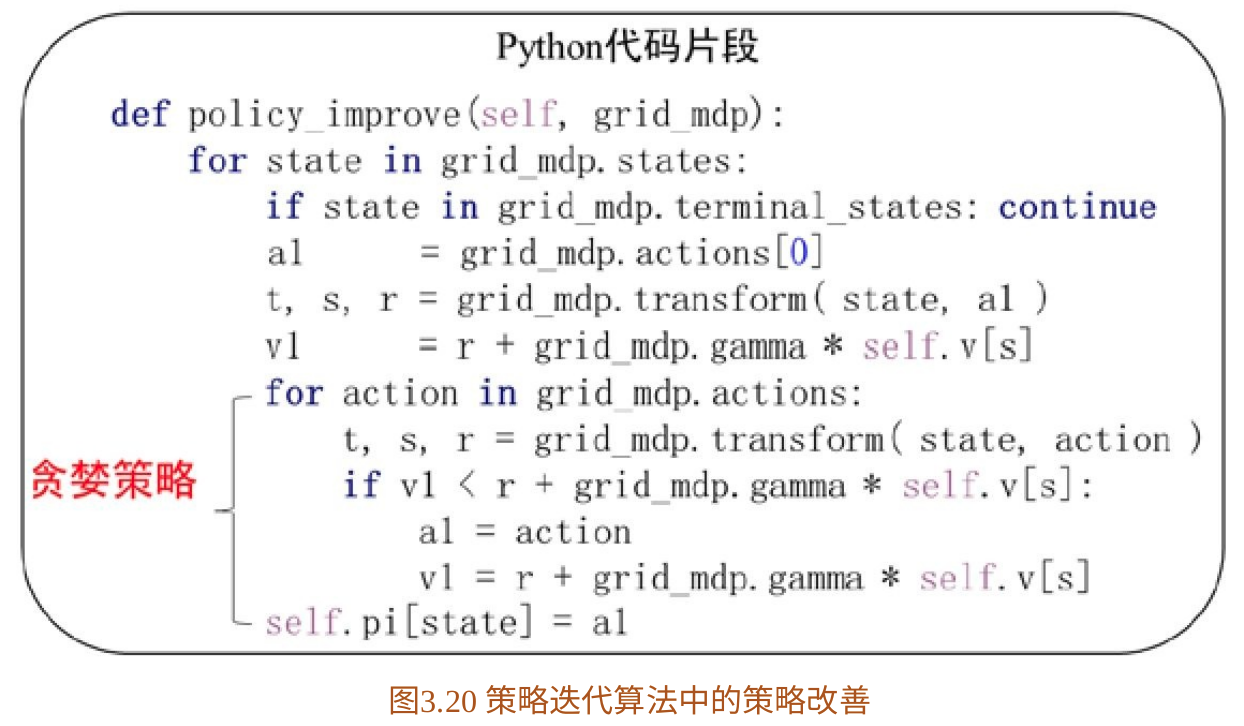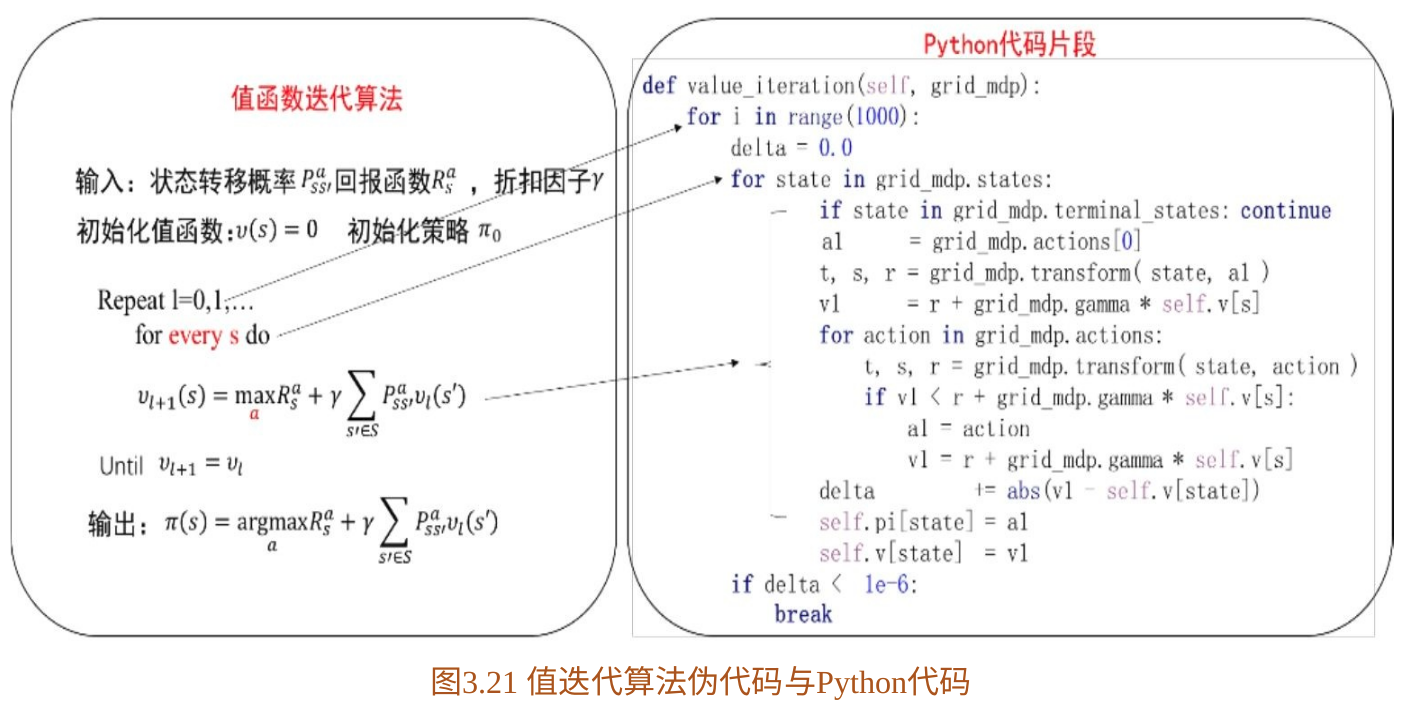## 6. 强化学习与最优控制

最优控制领域经过几十年的发展有许多的优秀成果，在基于模型的强化学习算法中可以利用这些优秀成果。基于模型的算法中，会先根据数据拟合出一个模型，根据已知的模型，最优控制领域中有很多好的方法可以计算出最优策略解，智能体再根据这些最优控制策略与环境交互，完成进一步优化。

结合最优控制的强化学习算法最典型的应用就是引导策略搜索算法。

展开全文强化学习 策略迭代算法 策略评估算法
• 强化学习有两种常见迭代训练算法：策略迭代算法和值迭代算法。在上一篇博客&amp;lt;&amp;lt;强化学习笔记（二）&amp;gt;&amp;gt;中已经详细描述了策略迭代算法，其实值迭代算法和策略迭代算法的基本...

强化学习有两种常见迭代训练算法：策略迭代算法和值迭代算法。在上一篇博客<<强化学习笔记（二）>>中已经详细描述了策略迭代算法，其实值迭代算法和策略迭代算法的基本思想是一致的，其最大的区别在于，策略迭代算法在进行策略改善的时候，使用的每个状态的值函数，是稳定的，在进行策略评估的时候，计算得到了当前策略的稳定值函数；而值迭代算法交替进行策略评估和策略改善的过程，并不是等到值函数稳定的时候再进行策略改善，其过程更为动态。

#### gym的样例代码展示

通过对下面的问题进行编程，加深对值迭代算法的理解。问题的描述同<<强化学习笔记(二)>>中的内容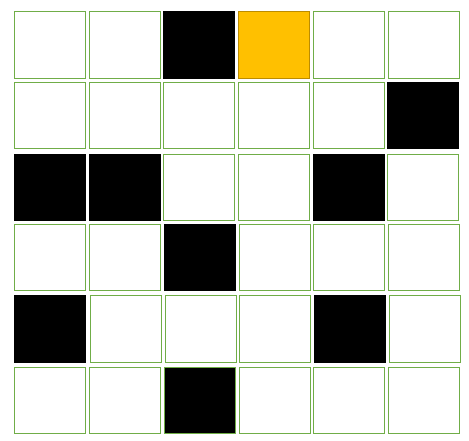该图的状态空间由下置上，从左到右，分别为1 – 36

import numpy as np
import random
from gym import spaces
import gym
from gym.envs.classic_control import rendering

#模拟环境类
class GridWorldEnv(gym.Env):
#相关的全局配置
'render.modes':['human', 'rgb_array'],
'video.frames_per_second': 2
}

def __init__(self):
self.states = [i for i in range(1, 37)] #初始化状态
self.terminate_states = [3, 7, 11, 15, 19, 20, 23, 30,  33, 34] #终结态
self.actions = ['up', 'down', 'left', 'right'] #动作空间

self.v_states = dict() #状态的值空间
for state in self.states:
self.v_states[state] = 0.0

for state in self.terminate_states: #先将所有陷阱和黄金的值函数初始化为-1.0
self.v_states[state] = -1.0

self.v_states = 1.0  #黄金的位置值函数初始化为 1

self.initStateAction() #初始化每个状态的可行动作空间
self.initStatePolicyAction() #随机初始化当前策略

self.gamma = 0.8 #计算值函数用的折扣因子
self.viewer = None #视图对象
self.current_state = None #当前状态
return

def translateStateToRowCol(self, state):
"""
将状态转化为行列坐标返回
"""
row = (state - 1) // 6
col = (state - 1) %  6
return row, col

def translateRowColToState(self, row, col):
"""
将行列坐标转化为状态值
"""
return row * 6 + col + 1

def actionRowCol(self, row, col, action):
"""
对行列坐标执行动作action并返回坐标
"""
if action == "up":
row = row - 1
if action == "down":
row = row + 1
if action == "left":
col = col - 1
if action == "right":
col = col + 1
return row, col

def canUp(self, row, col):
row = row - 1
return 0 <= row <= 5

def canDown(self, row, col):
row = row + 1
return 0 <= row <= 5

def canLeft(self, row, col):
col = col - 1
return 0 <= col <= 5

def canRight(self, row, col):
col = col + 1
return 0 <= col <= 5

def initStateAction(self):
"""
初始化每个状态可行动作空间
"""
self.states_actions = dict()
for state in self.states:
self.states_actions[state] = []
if state in self.terminate_states:
continue
row, col = self.translateStateToRowCol(state)
if self.canUp(row, col):
self.states_actions[state].append("up")
if self.canDown(row, col):
self.states_actions[state].append("down")
if self.canLeft(row, col):
self.states_actions[state].append('left')
if self.canRight(row, col):
self.states_actions[state].append('right')
return

def initStatePolicyAction(self):
"""
初始化每个状态的当前策略动作
"""
self.states_policy_action = dict()
for state in self.states:
if state in self.terminate_states:
self.states_policy_action[state] = None
else:
self.states_policy_action[state] = random.sample(self.states_actions[state], 1)
return

def seed(self, seed = None):
random.seed(seed)
return [seed]

def reset(self):
"""
重置原始状态
"""
self.current_state = random.sample(self.states, 1)

def step(self, action):
"""
动作迭代函数
"""
cur_state = self.current_state
if cur_state in self.terminate_states:
return cur_state, 0, True, {}
row, col = self.translateStateToRowCol(cur_state)
n_row, n_col = self.actionRowCol(row, col, action)
next_state = self.translateRowColToState(n_row, n_col)
self.current_state = next_state
if next_state in self.terminate_states:
return next_state, 0, True, {}
else:
return next_state, 0, False, {}

def policy_evaluate_and_improve(self):
"""
遍历状态空间，对策略进行评估和改善
"""
error = 0.0 #迭代的值函数误差
for state in self.states:
if state in self.terminate_states:
continue
action = self.states_policy_action[state]
self.current_state = state
next_state, reward, isTerminate, info = self.step(action)
new_value = reward + self.gamma * self.v_states[next_state]
new_action = action

for _action in self.states_actions[state]:
self.current_state = state
next_state, reward, isTerminate, info = self.step(_action)
if new_value < reward + self.v_states[next_state]:
new_value = reward + self.gamma * self.v_states[next_state]
new_action = _action
error = max(error, abs(new_value - self.v_states[state]))
self.v_states[state] = new_value
self.states_policy_action[state] = new_action
return error

def createGrids(self):
"""
创建网格
"""
start_x = 40
start_y = 40
line_length = 40
for state in self.states:
row, col = self.translateStateToRowCol(state)
x = start_x + col * line_length
y = start_y + row * line_length
line = rendering.Line((x, y), (x + line_length, y))
line.set_color(0, 0, 0)
line = rendering.Line((x, y), (x, y  + line_length))
line.set_color(0, 0, 0)
line = rendering.Line((x + line_length, y), (x + line_length, y  + line_length))
line.set_color(0, 0, 0)
line = rendering.Line((x, y + line_length), (x + line_length, y  + line_length))
line.set_color(0, 0, 0)

def createTraps(self):
"""
创建陷阱,将黄金的位置也先绘制成陷阱，后面覆盖画成黄金
"""
start_x = 40
start_y = 40
line_length = 40
for state in self.terminate_states:
row, col = self.translateStateToRowCol(state)
trap = rendering.make_circle(20)
trans = rendering.Transform()
trap.set_color(0, 0, 0)
trans.set_translation(start_x + line_length * col + 20, start_y + line_length * row + 20)

def createGold(self):
"""
创建黄金
"""
start_x = 40
start_y = 40
line_length = 40
state = 34
row, col = self.translateStateToRowCol(state)
gold = rendering.make_circle(20)
trans = rendering.Transform()
gold.set_color(1, 0.9, 0)
trans.set_translation(start_x + line_length * col + 20, start_y + line_length * row + 20)

def createRobot(self):
"""
创建机器人
"""
start_x = 40
start_y = 40
line_length = 40
row, col = self.translateStateToRowCol(self.current_state)
robot = rendering.make_circle(15)
trans = rendering.Transform()
robot.set_color(1, 0, 1)
trans.set_translation(start_x + line_length * col + 20, start_y + line_length * row + 20)

def render(self, mode="human", close=False):
"""
渲染整个场景
"""
#关闭视图
if close:
if self.viewer is not None:
self.viewer.close()
self.viewer = None

#视图的大小
screen_width = 320
screen_height = 320

if self.viewer is None:
self.viewer = rendering.Viewer(screen_width, screen_height)

#创建网格
self.createGrids()
#创建陷阱
self.createTraps()
#创建黄金
self.createGold()
#创建机器人
self.createRobot()
return self.viewer.render(return_rgb_array= mode == 'rgb_array')
##### 注册环境模拟类到gym
from gym.envs.registration import register
try:
register(id = "GridWorld-v4", entry_point=GridWorldEnv, max_episode_steps = 200, reward_threshold=100.0)
except:
pass
##### 进行策略迭代算法的过程和模拟动画的代码
from time import sleep
env = gym.make('GridWorld-v4')
env.reset()

#策略评估和策略改善
not_changed_count = 0
for _ in range(10000):
error = env.env.policy_evaluate_and_improve()
if error < 0.00001:
break
else:
#打印迭代的次数
print("iter count:" + str(_))

#观察env到底是个什么东西的打印信息。
print(isinstance(env, GridWorldEnv))
print(type(env))
print(env.__dict__)
print(isinstance(env.env, GridWorldEnv))

env.reset()

for _ in range(1000):
env.render()
if env.env.states_policy_action[env.env.current_state] is not None:
observation,reward,done,info = env.step(env.env.states_policy_action[env.env.current_state])
else:
done = True
print(_)
if done:
sleep(0.5)
env.render()
env.reset()
print("reset")
sleep(0.5)
env.close()
##### 动画效果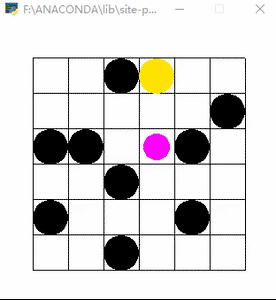展开全文• 基于点的值迭代算法是求解POMDP问题的一类近似解法。针对基于点的算法中信念选择这一关键问题,提出了一种基于熵的信念选择方法(EBBS).EBBS算法通过计算可以转移到的信念点的不确定性,选择熵较小且到当前信念点集距离...
• 强化学习算法-基于python的值迭代算法value-iteration实现
• 确定性清洁机器人的基于模型的值迭代算法。 这段代码是值迭代算法的一个非常简单的实现，对于强化学习和动态规划领域的初学者来说，它是一个有用的起点。 确定性清洁机器人 MDP：清洁机器人必须收集用过的罐子，还...matlab
•研究论文
• 网格世界模拟博弈中求解顺序决策问题的修正值迭代算法和路径规划 // Ganesh Rakate, Faris Al Afif // 电子邮件： , 该程序是基于java applet 的程序，需要使用浏览器启动。 使用浏览器打开 mazeapplet.html 文件...Java
• 基于循环卷积神经网络的POMDP值迭代算法.pdf
• 此代码以三维空间为例，编程实现了矩阵特征的幂迭代算法。可以扩展到任意维数。
• 【内容介绍】利用Rayleigh 商迭代法计算对称矩阵的特征
• jacobi雅克比迭代法求实对称矩阵的特征和特征向量
• 确定性清洁机器人的基于模型的策略迭代算法。 这段代码是策略迭代算法的一个非常简单的实现，对于强化学习和动态规划领域的初学者来说，它是一个有用的起点。 确定性清洁机器人 MDP：清洁机器人必须收集用过的罐子，...matlab
• 迭代法求指纹图像中的阀 [日期：2004-12-8] 来源：电子技术应用 作者：黎妹红 张其善 [字体：大 中 小] 　 摘要：给出使用直方图方法求阀的例子；结合直方图方法提出用于计算最优阀迭代法，该...
• 可以简单求解任何一个函数或者方程的牛顿迭代法的matlab程序
• 基于迭代法的自动阈值分割代码，用于matlab图像处理技术。
• 1.1 Jacobi迭代算法 def Jacobi_tensor_V2(A,b,Delta,m,n,M): start=time.perf_counter()#开始计时 find=0#用于标记是否在规定步数内收敛 X=np.ones(n)#迭代起始点 x=np.ones(n)#用于存储迭代的中间结果 d=np.ones...
• 利用Python，实现雅克比(Jacobi)迭代法以及高斯-塞德尔(G-S)迭代法【矩阵形式】 本文讲解使用Jacobi迭代和G-S迭代算法求解方程组的Python代码实现，同时涉及算法的原理阐述。 文章目录【Jacobi算法原理】【Jacobi的...
• 上一次已经分享了强化学习的概念以及基本的MDP，本节将分享基于Bellman方程和动态规划的策略迭代和值迭代，对于Bellman方程，大家都比较清楚了，那么我们先介绍一下动态规划算法的基本原理 一、动态规划 这里面我...

上一次已经分享了强化学习的概念以及基本的MDP，本节将分享基于Bellman方程和动态规划的策略迭代和值迭代，对于Bellman方程，大家都比较清楚了，那么我们先介绍一下动态规划算法的基本原理

一、动态规划

这里面我要简单介绍一下动态规划，因为严格来说，值迭代与策略迭代是用来解决动态规划问题的两种规划方法。而强化学习又有另外一个昵称——就是拟动态规划。说白了强化学习就是模拟动态规划算法。

用一句话来总结动态规划就是，对一个复杂问题给出一个一般性的解决办法。它主要由两个性质:

1、最优子结构：最优解法能被分解到多个子问题中

2、重叠子问题：子问题能重复多次且解法能被重复利用

马尔科夫决策过程（MDP）满足以上两个性质，所以任何MDP都可以用动态规划来解。动态规划与强化学习的区别就是动态规划假设MDP模型是全知的（即参数可知） 而强化学习可以使MDP未知。

MDP需要解决的问题有两种，第一种是prediction，它已知MDP的S,A,P,R,γ以及policy，目标是算出在每个状态下的value function(值函数其实就是问题的目标，一般都是跟reward有关的函数，例如Atari小游戏，一般值函数就是累计的得分的期望。目标一般就是最大化这个值函数。而第二种是control，它已知MDP的S,A,P,R,γ但是policy未知（即动作action未知），因此它的目标不仅是计算出最优的value function而且要给出最优的Policy。

二、策略迭代POLICY ITERATION

策略迭代就是在policy未知的情况下，根据每次的reward学到最优policy。策略迭代每次先初始化每个状态的值函数v(s)和一个策略，然后根据这个策略计算值函数v(s), 通过这个值函数来根据贪心策略更新策略，不断迭代直到策略收敛，下面是算法的流程：

1、initialization

初始化所有状态的v(s)以及π(s)（初始化为随机策略）

2、poicy evaluation

用当前的v(s)对当前策略进行评估，计算出每一个状态的v(s)，直到v(s)收敛，才算训练好了这个状态价值函数V(s)

3、policy improvement

既然上一步已经得到了当前策略的评估函数V(s),那么就可以利用这个评估函数进行策略改进啦。

在每个状态s时，对每个可能的动作a,都计算一下采取这个动作后到达的下一个状态的期望价值。看看哪个动作可以到达的状态的期望价值函数最大，就选取这个动作。以此更新了π(s)

然后再次循环上述２、３步骤，直到V(s)与π(s)都收敛。

下面总结一下Policy evaluation和Policy improvement这两个阶段：

2.1策略评估Policy evaluation

策略评估就是在任意策略下，计算每个状态的值函数vπ，我们也把它称为预测问题。由值函数的定义Vπ(s)=Eπ[GtSt=s]展开为

Vπ(s)=aπ(as)sP(ss,a)(R(s,a,s)+γVπ(s))

其中π(as)为在s状态下执行动作a的概率，

下面是策略评估的python代码

def policy_evaluation(self, agent, max_iter=-1):

iteration = 0

# iterative eval

while True:

# one iteration

iteration += 1

new_value_pi = agent.value_pi.copy()

for i in range(1, agent.s_len):  # for each state

value_sas = []

ac = agent.pi[i]

transition = agent.p[ac, i, :]

value_sa = np.dot(transition, agent.r + agent.gamma * agent.value_pi)

new_value_pi[i] = value_sa  # value_sas[agent.policy[i]]

diff = np.sqrt(np.sum(np.power(agent.value_pi - new_value_pi, 2)))

# print 'diff={}'.format(diff)

if diff < 1e-6:

break

else:

agent.value_pi = new_value_pi

if iteration == max_iter:

break

2.2策略改进Policy improvement

策略改进是Greedy policy，它寻找每个状态下得到最大值函数vπ的策略π，也就是说，采取哪个动作可以达到下一个状态的最大值，那么就选择这个动作为最优策略

计算期望值

Qπ(s,a)=s,rP(s,rs,a)(r+γVπ(s))

改进策略

π(s)=argmaxaQπ(s,a)

改进策略后更新状态值

Vπ(s)=maxas,rP(s,rs,a)(r+γVπ(s))

策略改进的python代码如下：

def policy_improvement(self, agent):

new_policy = np.zeros_like(agent.pi)

for i in range(1, agent.s_len):

for j in range(0, agent.a_len):

agent.value_q[i, j] = np.dot(agent.p[j, i, :], agent.r + agent.gamma * agent.value_pi)

max_act = np.argmax(agent.value_q[i, :])

new_policy[i] = max_act

if np.all(np.equal(new_policy, agent.pi)):

return False

else:

agent.pi = new_policy

return True

策略迭代算法的伪代码如下所示：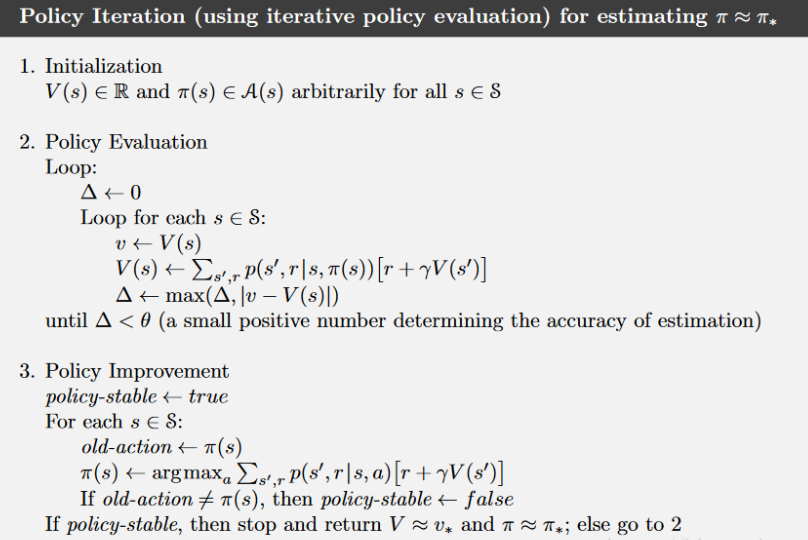三、价值迭代VALUE ITERATION

价值迭代算法主要流程如下。

1、初始化Value Function，即对每个状态的价值都赋一个初始值，一般是0

2、计算每一个状态-动作pair 的值，也就是Q(s,a)

3、 每一个状态st​下都有一个动作空间at​∈A，maxat​​Q(st​,at​)，即最大的Q值作为当前状态的价值，更新Value Function

4、返回第2步，直至Value Function收敛。

迭代公式如下：

Vt+1(s)=maxs,rP(s,rs,a)(r+γVt(s))

值迭代的伪代码如下：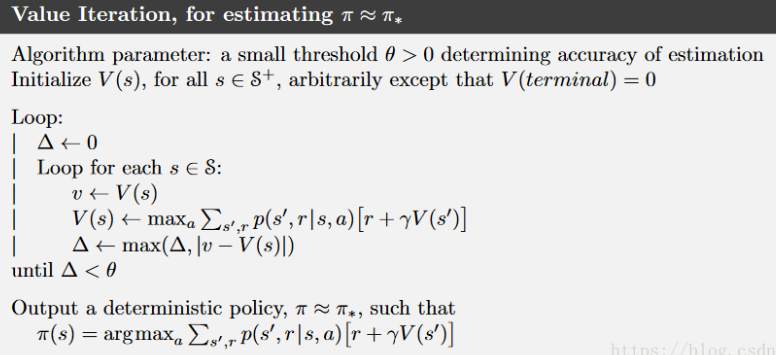值迭代的python代码如下：

def value_iteration(self, agent, max_iter=-1):

iteration = 0

while True:

iteration += 1

new_value_pi = np.zeros_like(agent.value_pi)

for i in range(1, agent.s_len):  # for each state

value_sas = []

for j in range(0, agent.a_len):  # for each act

value_sa = np.dot(agent.p[j, i, :], agent.r + agent.gamma * agent.value_pi)

value_sas.append(value_sa)

new_value_pi[i] = max(value_sas)

diff = np.sqrt(np.sum(np.power(agent.value_pi - new_value_pi, 2)))

if diff < 1e-6:

break

else:

agent.value_pi = new_value_pi

if iteration == max_iter:

break

print('Iter {} rounds converge'.format(iteration))

for i in range(1, agent.s_len):

for j in range(0, agent.a_len):

agent.value_q[i, j] = np.dot(agent.p[j, i, :], agent.r + agent.gamma * agent.value_pi)

max_act = np.argmax(agent.value_q[i, :])

agent.pi[i] = max_act

那么策略迭代和值迭代有什么区别和联系呢？

共同点：

1. 他们都是基于模型的方法，也就是说都需要知道环境的状态转移概率P；
2. 都需要通过bellman方程来更新状态值函数

不同点：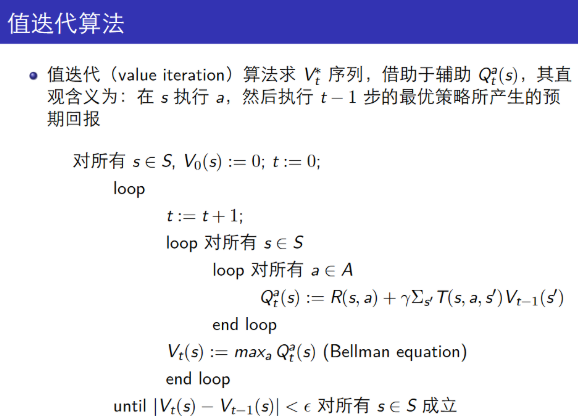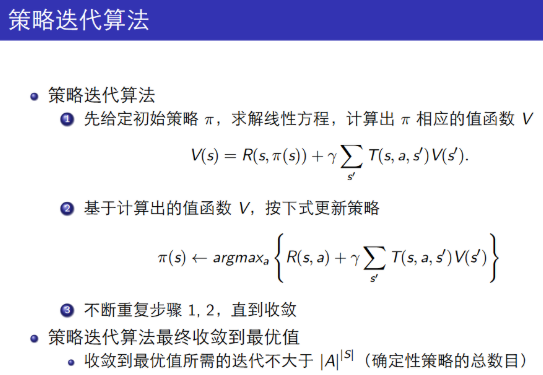1、策略迭代在价值评估阶段，每迭代一次都需要保证每个状态的值函数收敛，这是非常耗时的；而值迭代是采用动态规划的思想来收敛每个状态的值函数的。

2、策略迭代的第二步policy evaluation与值迭代的第二步finding optimal value function十分相似，除了后者用了max操作，前者没有max.因此后者可以得出optimal value function, 而前者不能得到optimal function.

3、策略迭代的收敛速度更快一些，在状态空间较小时，最好选用策略迭代方法。当状态空间较大时，值迭代的计算量更小一些。

4*****、侧重点不同：策略迭代最后是策略收敛，而值迭代是值函数收敛；收敛的方式也不同，策略迭代是argmax，而值函数是max

展开全文强化学习
• ## 策略迭代与值迭代的区别

万次阅读 多人点赞 2017-08-31 20:35:46
策略迭代与值迭代都属于强化学习里面策略求解中的动态规划方法。其区别是什么呢？ 首先看一张图片： 首先看策略迭代： １.initialization 初始化所有状态的v(s)以及π(s)（初始化为随机策略） 2....强化学习
• 【分析】 求平方根的迭代公式：x1 = 1/2 × ...（3）再次利用迭代公式求出一个新的x1，比较x1和x0，如果它们的差值小于指定的EPS（假设为1e-6），该非常趋近于真正的平方根，x1可以作为平方根的近似；否则将x...
• 求解线性⽅方程组 Ax=b，其中 A 为 ...比较 Jacobi 迭代法、Gauss-Seidel 迭代法、逐次超松弛迭代法、 共轭梯度法与高斯消去法、列主元消去法的计算时间。改变逐次超松弛迭代法的松弛因⼦， 分析其对收敛速度的影响。...